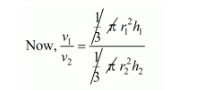# If the volumes of two cones are in the ratio 1 : 4`
Question:

If the volumes of two cones are in the ratio 1 : 4 and their diameters are in the ratio 4 : 5, then write the ratio of their weights.

Solution:

The ratio of the volume of cones

$v_{1}: v_{2}=1: 4$

And $2 r_{1}: 2 r_{2}=4: 5$,

i.e., $\frac{r_{1}}{r_{2}}=\frac{4}{5}$$\frac{1}{4}=\left(\frac{r_{1}}{r_{2}}\right)^{2} \times\left(\frac{h_{1}}{h_{2}}\right)$

$\frac{h_{1}}{h_{2}}=\frac{1}{4} \times \frac{25}{16}$

$=\frac{25}{64}$

Hence, $h_{1}: h_{2}=25: 64$